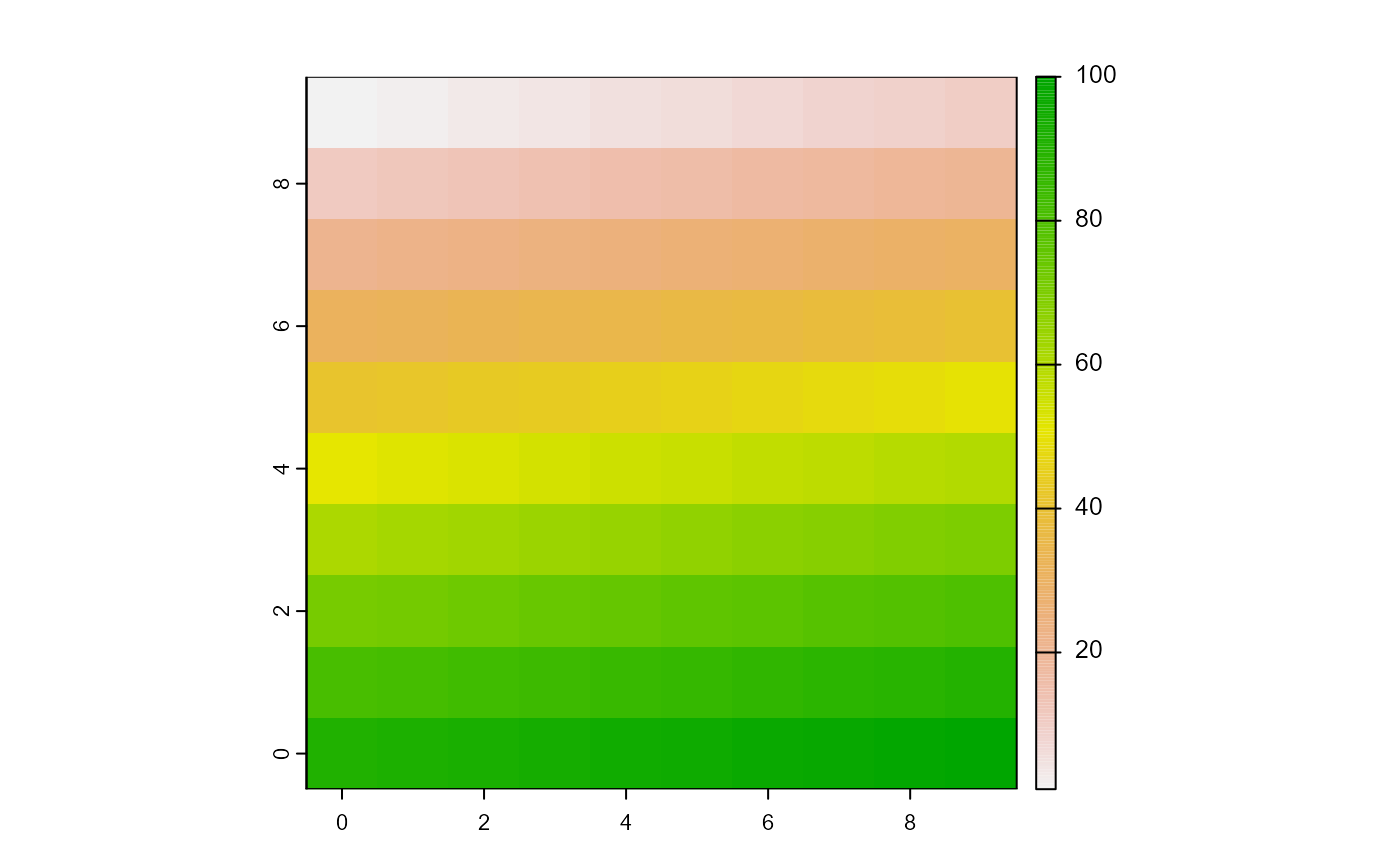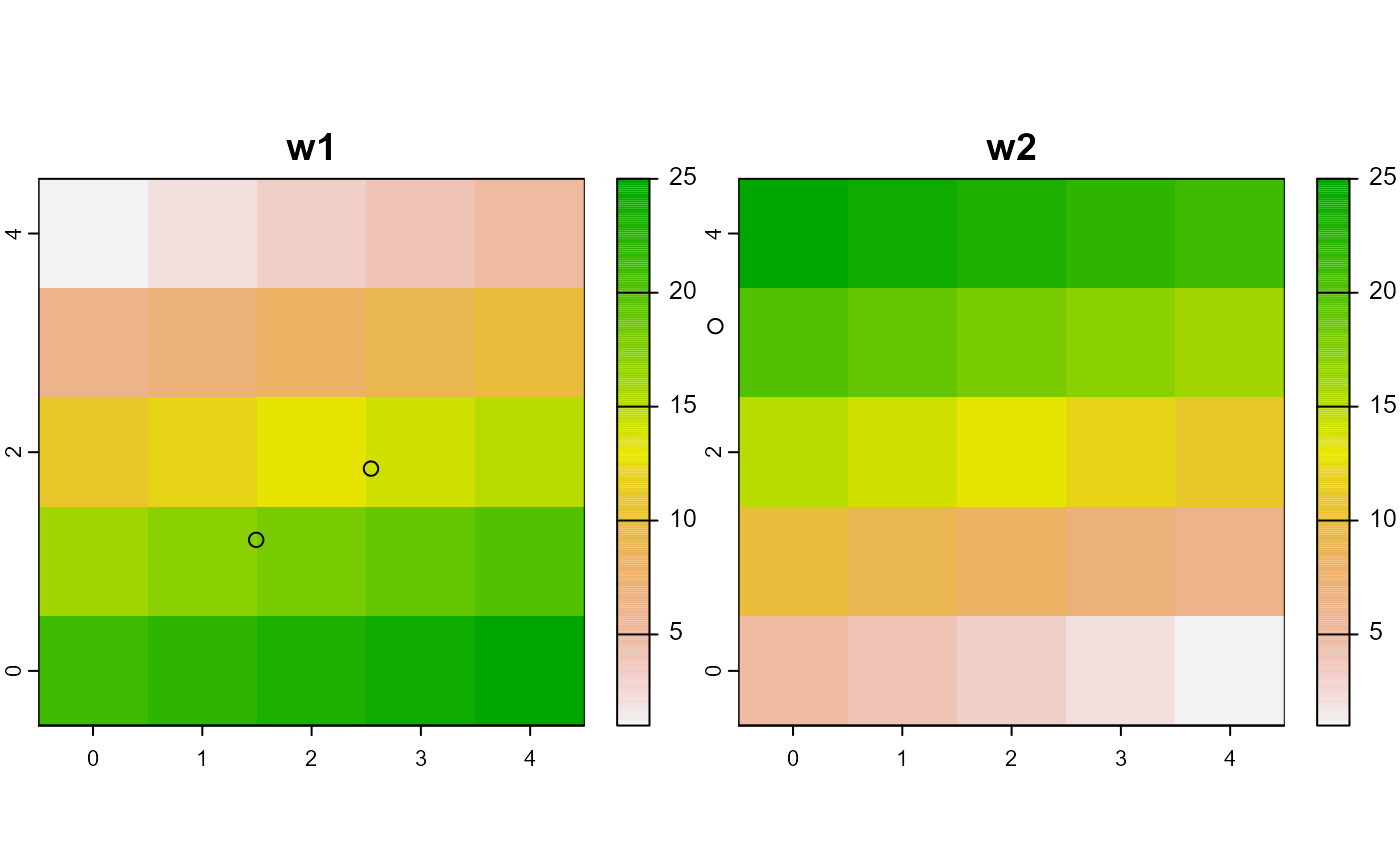These pass to plot, as a matrix of points (agentMatrix), as a raster (worldMatrix), or a rasterStack (worldArray). They can be modified.

# S3 method for agentMatrix
plot(x, ...)

# S3 method for worldMatrix
plot(x, ...)

# S3 method for worldArray
plot(x, ...)

# S3 method for agentMatrix
points(x, ...)

## Arguments

x an agentMatrix, worldMatrix or worldArray object arguments passed to plot methods for matrix (agentMatrix) or raster (world*)

## Examples

# agentMatrix
newAgent <- new("agentMatrix",
coords = cbind(pxcor = c(1, 2, 5), pycor = c(3, 4, 6)),
char = letters[c(1, 2, 6)],
nums2 = c(4.5, 2.6, 2343),
char2 = LETTERS[c(4, 24, 3)],
nums = 5:7)
plot(newAgent)## worldMatrix
w1 <- createWorld(minPxcor = 0, maxPxcor = 9, minPycor = 0, maxPycor = 9, data = 1:100)
plot(w1)## worldArray
w1 <- createWorld(minPxcor = 0, maxPxcor = 4, minPycor = 0, maxPycor = 4, data = 1:25)
w2 <- createWorld(minPxcor = 0, maxPxcor = 4, minPycor = 0, maxPycor = 4, data = 25:1)
w3 <- stackWorlds(w1, w2)
plot(w3)
# agentMatrix
newAgent <- new("agentMatrix",
coords = cbind(pxcor = c(1, 2, 5), pycor = c(3, 4, 6)),
char = letters[c(1, 2, 6)],
nums2 = c(4.5, 2.6, 2343),
char2 = LETTERS[c(4, 24, 3)],
nums = 5:7)
points(newAgent)